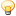CodeGuru Home VC++ / MFC / C++ .NET / C# Visual Basic VB Forums Developer.com

# Thread: Set Covering : Runtime hang\error at function call in c

1.Junior MemberJoin Date
Nov 2011
Posts
1

##Set Covering : Runtime hang\error at function call in c

I am implementing a set covering application which uses cover function

int cover(set *skill_list,set *player_list,set *covering)
Suppose skill_set={a,b,c,d,e}, player_list={s1,s2,s3} then output coverin ={s1,s3} where say s1={a,b,c}, s3={d,e} and s2={b,d}.

Now when I am calling this function it's hanging at run (set_cover.exe stopped working).

Here is my cover function:

typedef struct Spst_{
void *key;
set *st;
}Spst;

int cover(set *skill_list,set *player_list,set *covering)
{
Liste *member,*max_member;
Spst *subset;
set *intersection;
void **data;
int max_size;

set_init(covering); //to initialize set covering initially

while(skill_list->size>0&&player_list->size>0)
{
max_size=0;
{
if(set_intersection(intersection,((Spst *)(member->data))->st,skill_list)!=0)
return -1;

if(intersection->size>max_size)
{
max_member=member;
max_size=intersection->size;
}

set_destroy(intersection); //at the end of iteration
}

if(max_size==0) //to check for no covering
return -1;

subset=(Spst *)max_member->data; //to insert max subset from play list to covering set
set_inselem(covering,subset);

for(member=(((Spst *)max_member->data)->st->head);member!=NULL;member=member->next) //to rem elem from skill list
{
data=(void **)member->data;
set_remelem(skill_list,data);
}

set_remelem(player_list,(void **)subset); //to rem subset from set of subsets play list
}

if(skill_list->size>0)
return -1;

return 0;
}

Now assuming I have defined three set type sets(as stated above) and calling from main as

cover(skills,subsets,covering);=> runtime hang Here
Please give inputs on the missing link in this or the prerequisites for a proper call to this function type required.

Assume other functions used in cover are tested and working fine.Reply With Quote

2.Junior MemberJoin Date
Nov 2011
Posts
6

## Re: Set Covering : Runtime hang\error at function call in c

Hi , you have not given the complete code as
set_intersection(intersection,((Spst *)(member->data))->st,skill_list);
function what it do we dont know ..infact i am not getting the idea about what the whole function cover is doing .. one more thing .. why using so many pointer .Reply With Quote

3.Elite Member Power PosterJoin Date
Apr 1999
Posts
27,449

## Re: Set Covering : Runtime hang\error at function call in cOriginally Posted by bitmeI am implementing a set covering application which uses cover function

int cover(set *skill_list,set *player_list,set *covering)
Suppose skill_set={a,b,c,d,e}, player_list={s1,s2,s3} then output coverin ={s1,s3} where say s1={a,b,c}, s3={d,e} and s2={b,d}.

Now when I am calling this function it's hanging at run (set_cover.exe stopped working).
You are using pointers all over the place in your code. By doing this, it is assumed you know how to debug a problem when you get a pointer related problem. So have you debugged your code?
Assume other functions used in cover are tested and working fine.
No. We cannot just assume that the other functions are working.

When you write a C program that is highly based on pointers, a "working" program may not be working at all. It could be corrupting memory, and you wouldn't know it.

To properly diagnose these issues, you must provide all your code to us, not just a few functions. We would then need to run your program on our machine to duplicate the problem. Trying to debug a program that has pointers like this is not possible with just a few functions being shown us and we are just to look at them.

But the bottom line is that you wrote this program, therefore you should be able to debug it yourself. Otherwise, don't undertake writing such complex and hard-to-maintain functions.

Code:
```typedef struct Spst_{
void *key;
set *st;
}Spst;

int cover(set *skill_list,set *player_list,set *covering)
{
Liste *member,*max_member;
Spst *subset;
set *intersection;
void **data;
int max_size;

set_init(covering);         //to initialize set covering initially

while(skill_list->size>0&&player_list->size>0)
{
max_size=0;
{
if(set_intersection(intersection,((Spst *)(member->data))->st,skill_list)!=0)
return -1;

if(intersection->size>max_size)
{
max_member=member;
max_size=intersection->size;
}

set_destroy(intersection);              //at the end of iteration
}

if(max_size==0)                 //to check for no covering
return -1;

subset=(Spst *)max_member->data;            //to insert max subset from play list to covering set
set_inselem(covering,subset);

for(member=(((Spst *)max_member->data)->st->head);member!=NULL;member=member->next)     //to rem elem from skill list
{
data=(void **)member->data;
set_remelem(skill_list,data);
}

set_remelem(player_list,(void **)subset);           //to rem subset from set of subsets play list
}

if(skill_list->size>0)
return -1;

return 0;
}```
That is an example of using code tags.

Regards,

Paul McKenzieReply With Quote

runtime, set####Posting Permissions

• You may not post new threads
• You may not post replies
• You may not post attachments
• You may not edit your posts
•